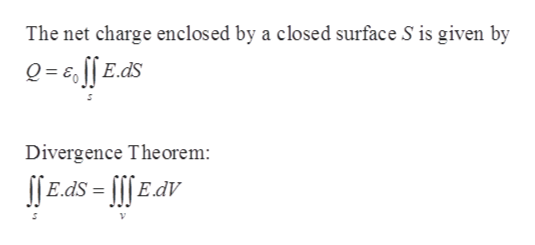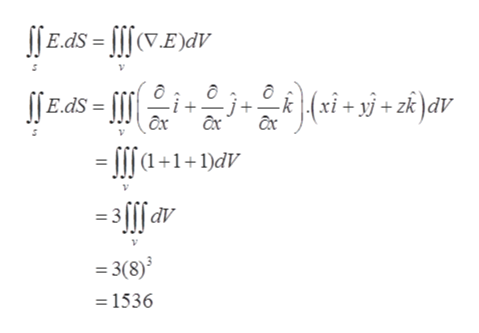# Use Gauss's Law to find the charge enclosed by the cube with vertices (±4, ±4, ±4) if the electric field is E(x, y, z) = x i + y j + z k.

Question
71 views

Use Gauss's Law to find the charge enclosed by the cube with vertices (±4, ±4, ±4) if the electric field is

E(x, y, z) = x i + y j + z k.
check_circle

Step 1

Given:

Step 2

Formula Used:help_outlineImage TranscriptioncloseThe net charge enclosed by a closed surface S is given by Q= 6, [[ E.ds Divergence Theorem: ſE.ds = [[[EaV fullscreen
Step 3

The Charge is giv...help_outlineImage TranscriptioncloseSſE.dS = [[[(V.E)dv ljEdS = [| = [[[(a +1+1)dV j+E(xi+ »j + zk }aV = 3[[[av dV = 3(8) = 1536 fullscreen

### Want to see the full answer?

See Solution

#### Want to see this answer and more?

Solutions are written by subject experts who are available 24/7. Questions are typically answered within 1 hour.*

See Solution
*Response times may vary by subject and question.
Tagged in

### Integration